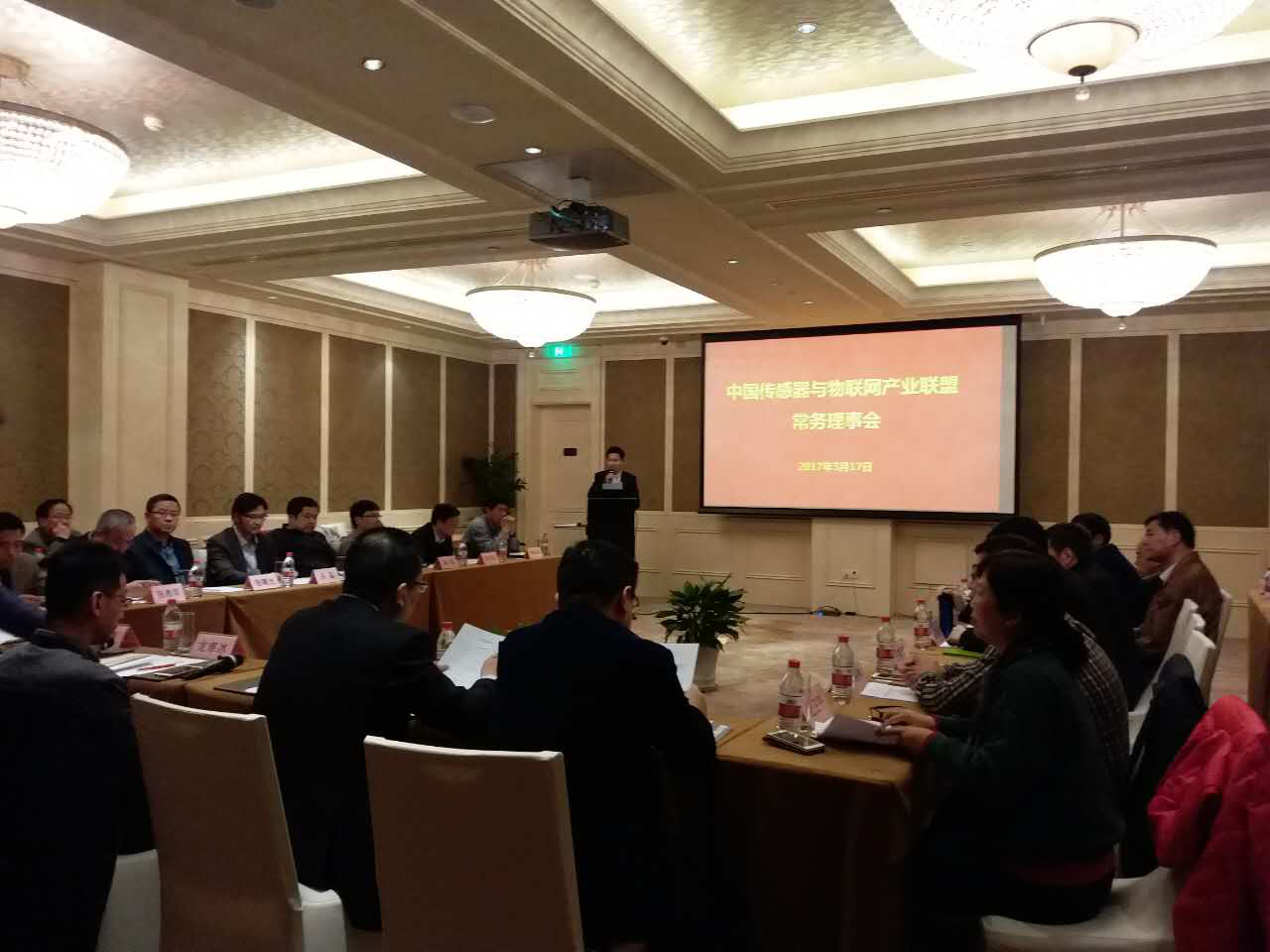t
",r.firstChild.style.width="5px",t.shrinkWrapBlocks=r.offsetWidth!==3,n.style.zoom=1),a.removeChild(n),n=r=s=o=null}),a.removeChild(p),n=r=s=o=u=a=p=null,t}();var D=/(?:\{[\s\S]*\}|\[[\s\S]*\])\$/,P=/([A-Z])/g;v.extend({cache:{},deletedIds:[],uuid:0,expando:"jQuery"+(v.fn.jquery+Math.random()).replace(/\D/g,""),noData:{embed:!0,object:"clsid:D27CDB6E-AE6D-11cf-96B8-444553540000",applet:!0},hasData:function(e){return e=e.nodeType?v.cache[e[v.expando]]:e[v.expando],!!e&&!B(e)},data:function(e,n,r,i){if(!v.acceptData(e))return;var s,o,u=v.expando,a=typeof n=="string",f=e.nodeType,l=f?v.cache:e,c=f?e[u]:e[u]&&u;if((!c||!l[c]||!i&&!l[c].data)&&a&&r===t)return;c||(f?e[u]=c=v.deletedIds.pop()||v.guid++:c=u),l[c]||(l[c]={},f||(l[c].toJSON=v.noop));if(typeof n=="object"||typeof n=="function")i?l[c]=v.extend(l[c],n):l[c].data=v.extend(l[c].data,n);return s=l[c],i||(s.data||(s.data={}),s=s.data),r!==t&&(s[v.camelCase(n)]=r),a?(o=s[n],o==null&&(o=s[v.camelCase(n)])):o=s,o},removeData:function(e,t,n){if(!v.acceptData(e))return;var r,i,s,o=e.nodeType,u=o?v.cache:e,a=o?e[v.expando]:v.expando;if(!u[a])return;if(t){r=n?u[a]:u[a].data;if(r){v.isArray(t)||(t in r?t=[t]:(t=v.camelCase(t),t in r?t=[t]:t=t.split(" ")));for(i=0,s=t.length;i1,null,!1))},removeData:function(e){return this.each(function(){v.removeData(this,e)})}}),v.extend({queue:function(e,t,n){var r;if(e)return t=(t||"fx")+"queue",r=v._data(e,t),n&&(!r||v.isArray(n)?r=v._data(e,t,v.makeArray(n)):r.push(n)),r||[]},dequeue:function(e,t){t=t||"fx";var n=v.queue(e,t),r=n.length,i=n.shift(),s=v._queueHooks(e,t),o=function(){v.dequeue(e,t)};i==="inprogress"&&(i=n.shift(),r--),i&&(t==="fx"&&n.unshift("inprogress"),delete s.stop,i.call(e,o,s)),!r&&s&&s.empty.fire()},_queueHooks:function(e,t){var n=t+"queueHooks";return v._data(e,n)||v._data(e,n,{empty:v.Callbacks("once memory").add(function(){v.removeData(e,t+"queue",!0),v.removeData(e,n,!0)})})}}),v.fn.extend({queue:function(e,n){var r=2;return typeof e!="string"&&(n=e,e="fx",r--),arguments.length1)},removeAttr:function(e){return this.each(function(){v.removeAttr(this,e)})},prop:function(e,t){return v.access(this,v.prop,e,t,arguments.length>1)},removeProp:function(e){return e=v.propFix[e]||e,this.each(function(){try{this[e]=t,delete this[e]}catch(n){}})},addClass:function(e){var t,n,r,i,s,o,u;if(v.isFunction(e))return this.each(function(t){v(this).addClass(e.call(this,t,this.className))});if(e&&typeof e=="string"){t=e.split(y);for(n=0,r=this.length;n=0)r=r.replace(" "+n[s]+" "," ");i.className=e?v.trim(r):""}}}return this},toggleClass:function(e,t){var n=typeof e,r=typeof t=="boolean";return v.isFunction(e)?this.each(function(n){v(this).toggleClass(e.call(this,n,this.className,t),t)}):this.each(function(){if(n==="string"){var i,s=0,o=v(this),u=t,a=e.split(y);while(i=a[s++])u=r?u:!o.hasClass(i),o[u?"addClass":"removeClass"](i)}else if(n==="undefined"||n==="boolean")this.className&&v._data(this,"__className__",this.className),this.className=this.className||e===!1?"":v._data(this,"__className__")||""})},hasClass:function(e){var t=" "+e+" ",n=0,r=this.length;for(;n=0)return!0;return!1},val:function(e){var n,r,i,s=this;if(!arguments.length){if(s)return n=v.valHooks[s.type]||v.valHooks[s.nodeName.toLowerCase()],n&&"get"in n&&(r=n.get(s,"value"))!==t?r:(r=s.value,typeof r=="string"?r.replace(R,""):r==null?"":r);return}return i=v.isFunction(e),this.each(function(r){var s,o=v(this);if(this.nodeType!==1)return;i?s=e.call(this,r,o.val()):s=e,s==null?s="":typeof s=="number"?s+="":v.isArray(s)&&(s=v.map(s,function(e){return e==null?"":e+""})),n=v.valHooks[this.type]||v.valHooks[this.nodeName.toLowerCase()];if(!n||!("set"in n)||n.set(this,s,"value")===t)this.value=s})}}),v.extend({valHooks:{option:{get:function(e){var t=e.attributes.value;return!t||t.specified?e.value:e.text}},select:{get:function(e){var t,n,r=e.options,i=e.selectedIndex,s=e.type==="select-one"||i<0,o=s?null:[],u=s?i+1:r.length,a=i<0?u:s?i:0;for(;a=0}),n.length||(e.selectedIndex=-1),n}}},attrFn:{},attr:function(e,n,r,i){var s,o,u,a=e.nodeType;if(!e||a===3||a===8||a===2)return;if(i&&v.isFunction(v.fn[n]))return v(e)[n](r);if(typeof e.getAttribute=="undefined")return v.prop(e,n,r);u=a!==1||!v.isXMLDoc(e),u&&(n=n.toLowerCase(),o=v.attrHooks[n]||(X.test(n)?F:j));if(r!==t){if(r===null){v.removeAttr(e,n);return}return o&&"set"in o&&u&&(s=o.set(e,r,n))!==t?s:(e.setAttribute(n,r+""),r)}return o&&"get"in o&&u&&(s=o.get(e,n))!==null?s:(s=e.getAttribute(n),s===null?t:s)},removeAttr:function(e,t){var n,r,i,s,o=0;if(t&&e.nodeType===1){r=t.split(y);for(;o=0}})});var \$=/^(?:textarea|input|select)\$/i,J=/^([^\.]*|)(?:\.(.+)|)\$/,K=/(?:^|\s)hover(\.\S+|)\b/,Q=/^key/,G=/^(?:mouse|contextmenu)|click/,Y=/^(?:focusinfocus|focusoutblur)\$/,Z=function(e){return v.event.special.hover?e:e.replace(K,"mouseenter\$1 mouseleave\$1")};v.event={add:function(e,n,r,i,s){var o,u,a,f,l,c,h,p,d,m,g;if(e.nodeType===3||e.nodeType===8||!n||!r||!(o=v._data(e)))return;r.handler&&(d=r,r=d.handler,s=d.selector),r.guid||(r.guid=v.guid++),a=o.events,a||(o.events=a={}),u=o.handle,u||(o.handle=u=function(e){return typeof v=="undefined"||!!e&&v.event.triggered===e.type?t:v.event.dispatch.apply(u.elem,arguments)},u.elem=e),n=v.trim(Z(n)).split(" ");for(f=0;f=0&&(y=y.slice(0,-1),a=!0),y.indexOf(".")>=0&&(b=y.split("."),y=b.shift(),b.sort());if((!s||v.event.customEvent[y])&&!v.event.global[y])return;n=typeof n=="object"?n[v.expando]?n:new v.Event(y,n):new v.Event(y),n.type=y,n.isTrigger=!0,n.exclusive=a,n.namespace=b.join("."),n.namespace_re=n.namespace?new RegExp("(^|\\.)"+b.join("\\.(?:.*\\.|)")+"(\\.|\$)"):null,h=y.indexOf(":")<0?"on"+y:"";if(!s){u=v.cache;for(f in u)u[f].events&&u[f].events[y]&&v.event.trigger(n,r,u[f].handle.elem,!0);return}n.result=t,n.target||(n.target=s),r=r!=null?v.makeArray(r):[],r.unshift(n),p=v.event.special[y]||{};if(p.trigger&&p.trigger.apply(s,r)===!1)return;m=[[s,p.bindType||y]];if(!o&&!p.noBubble&&!v.isWindow(s)){g=p.delegateType||y,l=Y.test(g+y)?s:s.parentNode;for(c=s;l;l=l.parentNode)m.push([l,g]),c=l;c===(s.ownerDocument||i)&&m.push([c.defaultView||c.parentWindow||e,g])}for(f=0;f=0:v.find(h,this,null,[s]).length),u[h]&&f.push(c);f.length&&w.push({elem:s,matches:f})}d.length>m&&w.push({elem:this,matches:d.slice(m)});for(r=0;r0?this.on(t,null,e,n):this.trigger(t)},Q.test(t)&&(v.event.fixHooks[t]=v.event.keyHooks),G.test(t)&&(v.event.fixHooks[t]=v.event.mouseHooks)}),function(e,t){function nt(e,t,n,r){n=n||[],t=t||g;var i,s,a,f,l=t.nodeType;if(!e||typeof e!="string")return n;if(l!==1&&l!==9)return[];a=o(t);if(!a&&!r)if(i=R.exec(e))if(f=i){if(l===9){s=t.getElementById(f);if(!s||!s.parentNode)return n;if(s.id===f)return n.push(s),n}else if(t.ownerDocument&&(s=t.ownerDocument.getElementById(f))&&u(t,s)&&s.id===f)return n.push(s),n}else{if(i)return S.apply(n,x.call(t.getElementsByTagName(e),0)),n;if((f=i)&&Z&&t.getElementsByClassName)return S.apply(n,x.call(t.getElementsByClassName(f),0)),n}return vt(e.replace(j,"\$1"),t,n,r,a)}function rt(e){return function(t){var n=t.nodeName.toLowerCase();return n==="input"&&t.type===e}}function it(e){return function(t){var n=t.nodeName.toLowerCase();return(n==="input"||n==="button")&&t.type===e}}function st(e){return N(function(t){return t=+t,N(function(n,r){var i,s=e([],n.length,t),o=s.length;while(o--)n[i=s[o]]&&(n[i]=!(r[i]=n[i]))})})}function ot(e,t,n){if(e===t)return n;var r=e.nextSibling;while(r){if(r===t)return-1;r=r.nextSibling}return 1}function ut(e,t){var n,r,s,o,u,a,f,l=L[d][e+" "];if(l)return t?0:l.slice(0);u=e,a=[],f=i.preFilter;while(u){if(!n||(r=F.exec(u)))r&&(u=u.slice(r.length)||u),a.push(s=[]);n=!1;if(r=I.exec(u))s.push(n=new m(r.shift())),u=u.slice(n.length),n.type=r.replace(j," ");for(o in i.filter)(r=J[o].exec(u))&&(!f[o]||(r=f[o](r)))&&(s.push(n=new m(r.shift())),u=u.slice(n.length),n.type=o,n.matches=r);if(!n)break}return t?u.length:u?nt.error(e):L(e,a).slice(0)}function at(e,t,r){var i=t.dir,s=r&&t.dir==="parentNode",o=w++;return t.first?function(t,n,r){while(t=t[i])if(s||t.nodeType===1)return e(t,n,r)}:function(t,r,u){if(!u){var a,f=b+" "+o+" ",l=f+n;while(t=t[i])if(s||t.nodeType===1){if((a=t[d])===l)return t.sizset;if(typeof a=="string"&&a.indexOf(f)===0){if(t.sizset)return t}else{t[d]=l;if(e(t,r,u))return t.sizset=!0,t;t.sizset=!1}}}else while(t=t[i])if(s||t.nodeType===1)if(e(t,r,u))return t}}function ft(e){return e.length>1?function(t,n,r){var i=e.length;while(i--)if(!e[i](t,n,r))return!1;return!0}:e}function lt(e,t,n,r,i){var s,o=[],u=0,a=e.length,f=t!=null;for(;u-1&&(s[f]=!(o[f]=c))}}else g=lt(g===o?g.splice(d,g.length):g),i?i(null,o,g,a):S.apply(o,g)})}function ht(e){var t,n,r,s=e.length,o=i.relative[e.type],u=o||i.relative[" "],a=o?1:0,f=at(function(e){return e===t},u,!0),l=at(function(e){return T.call(t,e)>-1},u,!0),h=[function(e,n,r){return!o&&(r||n!==c)||((t=n).nodeType?f(e,n,r):l(e,n,r))}];for(;a1&&ft(h),a>1&&e.slice(0,a-1).join("").replace(j,"\$1"),n,a0,s=e.length>0,o=function(u,a,f,l,h){var p,d,v,m=[],y=0,w="0",x=u&&[],T=h!=null,N=c,C=u||s&&i.find.TAG("*",h&&a.parentNode||a),k=b+=N==null?1:Math.E;T&&(c=a!==g&&a,n=o.el);for(;(p=C[w])!=null;w++){if(s&&p){for(d=0;v=e[d];d++)if(v(p,a,f)){l.push(p);break}T&&(b=k,n=++o.el)}r&&((p=!v&&p)&&y--,u&&x.push(p))}y+=w;if(r&&w!==y){for(d=0;v=t[d];d++)v(x,m,a,f);if(u){if(y>0)while(w--)!x[w]&&!m[w]&&(m[w]=E.call(l));m=lt(m)}S.apply(l,m),T&&!u&&m.length>0&&y+t.length>1&&nt.uniqueSort(l)}return T&&(b=k,c=N),x};return o.el=0,r?N(o):o}function dt(e,t,n){var r=0,i=t.length;for(;r2&&(f=u).type==="ID"&&t.nodeType===9&&!s&&i.relative[u.type]){t=i.find.ID(f.matches.replace(\$,""),t,s);if(!t)return n;e=e.slice(u.shift().length)}for(o=J.POS.test(e)?-1:u.length-1;o>=0;o--){f=u[o];if(i.relative[l=f.type])break;if(c=i.find[l])if(r=c(f.matches.replace(\$,""),z.test(u.type)&&t.parentNode||t,s)){u.splice(o,1),e=r.length&&u.join("");if(!e)return S.apply(n,x.call(r,0)),n;break}}}return a(e,h)(r,t,s,n,z.test(e)),n}function mt(){}var n,r,i,s,o,u,a,f,l,c,h=!0,p="undefined",d=("sizcache"+Math.random()).replace(".",""),m=String,g=e.document,y=g.documentElement,b=0,w=0,E=[].pop,S=[].push,x=[].slice,T=[].indexOf||function(e){var t=0,n=this.length;for(;ti.cacheLength&&delete e[t.shift()],e[n+" "]=r},e)},k=C(),L=C(),A=C(),O="[\\x20\\t\\r\\n\\f]",M="(?:\\\\.|[-\\w]|[^\\x00-\\xa0])+",_=M.replace("w","w#"),D="([*^\$|!~]?=)",P="\\["+O+"*("+M+")"+O+"*(?:"+D+O+"*(?:(['\"])((?:\\\\.|[^\\\\])*?)\\3|("+_+")|)|)"+O+"*\\]",H=":("+M+")(?:\\((?:(['\"])((?:\\\\.|[^\\\\])*?)\\2|([^()[\\]]*|(?:(?:"+P+")|[^:]|\\\\.)*|.*))\\)|)",B=":(even|odd|eq|gt|lt|nth|first|last)(?:\\("+O+"*((?:-\\d)?\\d*)"+O+"*\\)|)(?=[^-]|\$)",j=new RegExp("^"+O+"+|((?:^|[^\\\\])(?:\\\\.)*)"+O+"+\$","g"),F=new RegExp("^"+O+"*,"+O+"*"),I=new RegExp("^"+O+"*([\\x20\\t\\r\\n\\f>+~])"+O+"*"),q=new RegExp(H),R=/^(?:#([\w\-]+)|(\w+)|\.([\w\-]+))\$/,U=/^:not/,z=/[\x20\t\r\n\f]*[+~]/,W=/:not\(\$/,X=/h\d/i,V=/input|select|textarea|button/i,\$=/\\(?!\\)/g,J={ID:new RegExp("^#("+M+")"),CLASS:new RegExp("^\\.("+M+")"),NAME:new RegExp("^\\[name=['\"]?("+M+")['\"]?\\]"),TAG:new RegExp("^("+M.replace("w","w*")+")"),ATTR:new RegExp("^"+P),PSEUDO:new RegExp("^"+H),POS:new RegExp(B,"i"),CHILD:new RegExp("^:(only|nth|first|last)-child(?:\\("+O+"*(even|odd|(([+-]|)(\\d*)n|)"+O+"*(?:([+-]|)"+O+"*(\\d+)|))"+O+"*\\)|)","i"),needsContext:new RegExp("^"+O+"*[>+~]|"+B,"i")},K=function(e){var t=g.createElement("div");try{return e(t)}catch(n){return!1}finally{t=null}},Q=K(function(e){return e.appendChild(g.createComment("")),!e.getElementsByTagName("*").length}),G=K(function(e){return e.innerHTML="",e.firstChild&&typeof e.firstChild.getAttribute!==p&&e.firstChild.getAttribute("href")==="#"}),Y=K(function(e){e.innerHTML="";var t=typeof e.lastChild.getAttribute("multiple");return t!=="boolean"&&t!=="string"}),Z=K(function(e){return e.innerHTML="",!e.getElementsByClassName||!e.getElementsByClassName("e").length?!1:(e.lastChild.className="e",e.getElementsByClassName("e").length===2)}),et=K(function(e){e.id=d+0,e.innerHTML="
",y.insertBefore(e,y.firstChild);var t=g.getElementsByName&&g.getElementsByName(d).length===2+g.getElementsByName(d+0).length;return r=!g.getElementById(d),y.removeChild(e),t});try{x.call(y.childNodes,0).nodeType}catch(tt){x=function(e){var t,n=[];for(;t=this[e];e++)n.push(t);return n}}nt.matches=function(e,t){return nt(e,null,null,t)},nt.matchesSelector=function(e,t){return nt(t,null,null,[e]).length>0},s=nt.getText=function(e){var t,n="",r=0,i=e.nodeType;if(i){if(i===1||i===9||i===11){if(typeof e.textContent=="string")return e.textContent;for(e=e.firstChild;e;e=e.nextSibling)n+=s(e)}else if(i===3||i===4)return e.nodeValue}else for(;t=e[r];r++)n+=s(t);return n},o=nt.isXML=function(e){var t=e&&(e.ownerDocument||e).documentElement;return t?t.nodeName!=="HTML":!1},u=nt.contains=y.contains?function(e,t){var n=e.nodeType===9?e.documentElement:e,r=t&&t.parentNode;return e===r||!!(r&&r.nodeType===1&&n.contains&&n.contains(r))}:y.compareDocumentPosition?function(e,t){return t&&!!(e.compareDocumentPosition(t)&16)}:function(e,t){while(t=t.parentNode)if(t===e)return!0;return!1},nt.attr=function(e,t){var n,r=o(e);return r||(t=t.toLowerCase()),(n=i.attrHandle[t])?n(e):r||Y?e.getAttribute(t):(n=e.getAttributeNode(t),n?typeof e[t]=="boolean"?e[t]?t:null:n.specified?n.value:null:null)},i=nt.selectors={cacheLength:50,createPseudo:N,match:J,attrHandle:G?{}:{href:function(e){return e.getAttribute("href",2)},type:function(e){return e.getAttribute("type")}},find:{ID:r?function(e,t,n){if(typeof t.getElementById!==p&&!n){var r=t.getElementById(e);return r&&r.parentNode?[r]:[]}}:function(e,n,r){if(typeof n.getElementById!==p&&!r){var i=n.getElementById(e);return i?i.id===e||typeof i.getAttributeNode!==p&&i.getAttributeNode("id").value===e?[i]:t:[]}},TAG:Q?function(e,t){if(typeof t.getElementsByTagName!==p)return t.getElementsByTagName(e)}:function(e,t){var n=t.getElementsByTagName(e);if(e==="*"){var r,i=[],s=0;for(;r=n[s];s++)r.nodeType===1&&i.push(r);return i}return n},NAME:et&&function(e,t){if(typeof t.getElementsByName!==p)return t.getElementsByName(name)},CLASS:Z&&function(e,t,n){if(typeof t.getElementsByClassName!==p&&!n)return t.getElementsByClassName(e)}},relative:{">":{dir:"parentNode",first:!0}," ":{dir:"parentNode"},"+":{dir:"previousSibling",first:!0},"~":{dir:"previousSibling"}},preFilter:{ATTR:function(e){return e=e.replace(\$,""),e=(e||e||"").replace(\$,""),e==="~="&&(e=" "+e+" "),e.slice(0,4)},CHILD:function(e){return e=e.toLowerCase(),e==="nth"?(e||nt.error(e),e=+(e?e+(e||1):2*(e==="even"||e==="odd")),e=+(e+e||e==="odd")):e&&nt.error(e),e},PSEUDO:function(e){var t,n;if(J.CHILD.test(e))return null;if(e)e=e;else if(t=e)q.test(t)&&(n=ut(t,!0))&&(n=t.indexOf(")",t.length-n)-t.length)&&(t=t.slice(0,n),e=e.slice(0,n)),e=t;return e.slice(0,3)}},filter:{ID:r?function(e){return e=e.replace(\$,""),function(t){return t.getAttribute("id")===e}}:function(e){return e=e.replace(\$,""),function(t){var n=typeof t.getAttributeNode!==p&&t.getAttributeNode("id");return n&&n.value===e}},TAG:function(e){return e==="*"?function(){return!0}:(e=e.replace(\$,"").toLowerCase(),function(t){return t.nodeName&&t.nodeName.toLowerCase()===e})},CLASS:function(e){var t=k[d][e+" "];return t||(t=new RegExp("(^|"+O+")"+e+"("+O+"|\$)"))&&k(e,function(e){return t.test(e.className||typeof e.getAttribute!==p&&e.getAttribute("class")||"")})},ATTR:function(e,t,n){return function(r,i){var s=nt.attr(r,e);return s==null?t==="!=":t?(s+="",t==="="?s===n:t==="!="?s!==n:t==="^="?n&&s.indexOf(n)===0:t==="*="?n&&s.indexOf(n)>-1:t==="\$="?n&&s.substr(s.length-n.length)===n:t==="~="?(" "+s+" ").indexOf(n)>-1:t==="|="?s===n||s.substr(0,n.length+1)===n+"-":!1):!0}},CHILD:function(e,t,n,r){return e==="nth"?function(e){var t,i,s=e.parentNode;if(n===1&&r===0)return!0;if(s){i=0;for(t=s.firstChild;t;t=t.nextSibling)if(t.nodeType===1){i++;if(e===t)break}}return i-=r,i===n||i%n===0&&i/n>=0}:function(t){var n=t;switch(e){case"only":case"first":while(n=n.previousSibling)if(n.nodeType===1)return!1;if(e==="first")return!0;n=t;case"last":while(n=n.nextSibling)if(n.nodeType===1)return!1;return!0}}},PSEUDO:function(e,t){var n,r=i.pseudos[e]||i.setFilters[e.toLowerCase()]||nt.error("unsupported pseudo: "+e);return r[d]?r(t):r.length>1?(n=[e,e,"",t],i.setFilters.hasOwnProperty(e.toLowerCase())?N(function(e,n){var i,s=r(e,t),o=s.length;while(o--)i=T.call(e,s[o]),e[i]=!(n[i]=s[o])}):function(e){return r(e,0,n)}):r}},pseudos:{not:N(function(e){var t=[],n=[],r=a(e.replace(j,"\$1"));return r[d]?N(function(e,t,n,i){var s,o=r(e,null,i,[]),u=e.length;while(u--)if(s=o[u])e[u]=!(t[u]=s)}):function(e,i,s){return t=e,r(t,null,s,n),!n.pop()}}),has:N(function(e){return function(t){return nt(e,t).length>0}}),contains:N(function(e){return function(t){return(t.textContent||t.innerText||s(t)).indexOf(e)>-1}}),enabled:function(e){return e.disabled===!1},disabled:function(e){return e.disabled===!0},checked:function(e){var t=e.nodeName.toLowerCase();return t==="input"&&!!e.checked||t==="option"&&!!e.selected},selected:function(e){return e.parentNode&&e.parentNode.selectedIndex,e.selected===!0},parent:function(e){return!i.pseudos.empty(e)},empty:function(e){var t;e=e.firstChild;while(e){if(e.nodeName>"@"||(t=e.nodeType)===3||t===4)return!1;e=e.nextSibling}return!0},header:function(e){return X.test(e.nodeName)},text:function(e){var t,n;return e.nodeName.toLowerCase()==="input"&&(t=e.type)==="text"&&((n=e.getAttribute("type"))==null||n.toLowerCase()===t)},radio:rt("radio"),checkbox:rt("checkbox"),file:rt("file"),password:rt("password"),image:rt("image"),submit:it("submit"),reset:it("reset"),button:function(e){var t=e.nodeName.toLowerCase();return t==="input"&&e.type==="button"||t==="button"},input:function(e){return V.test(e.nodeName)},focus:function(e){var t=e.ownerDocument;return e===t.activeElement&&(!t.hasFocus||t.hasFocus())&&!!(e.type||e.href||~e.tabIndex)},active:function(e){return e===e.ownerDocument.activeElement},first:st(function(){return}),last:st(function(e,t){return[t-1]}),eq:st(function(e,t,n){return[n<0?n+t:n]}),even:st(function(e,t){for(var n=0;n=0;)e.push(r);return e}),gt:st(function(e,t,n){for(var r=n<0?n+t:n;++r",e.querySelectorAll("[selected]").length||i.push("\\["+O+"*(?:checked|disabled|ismap|multiple|readonly|selected|value)"),e.querySelectorAll(":checked").length||i.push(":checked")}),K(function(e){e.innerHTML="

",e.querySelectorAll("[test^='']").length&&i.push("[*^\$]="+O+"*(?:\"\"|'')"),e.innerHTML="",e.querySelectorAll(":enabled").length||i.push(":enabled",":disabled")}),i=new RegExp(i.join("|")),vt=function(e,r,s,o,u){if(!o&&!u&&!i.test(e)){var a,f,l=!0,c=d,h=r,p=r.nodeType===9&&e;if(r.nodeType===1&&r.nodeName.toLowerCase()!=="object"){a=ut(e),(l=r.getAttribute("id"))?c=l.replace(n,"\\\$&"):r.setAttribute("id",c),c="[id='"+c+"'] ",f=a.length;while(f--)a[f]=c+a[f].join("");h=z.test(e)&&r.parentNode||r,p=a.join(",")}if(p)try{return S.apply(s,x.call(h.querySelectorAll(p),0)),s}catch(v){}finally{l||r.removeAttribute("id")}}return t(e,r,s,o,u)},u&&(K(function(t){e=u.call(t,"div");try{u.call(t,"[test!='']:sizzle"),s.push("!=",H)}catch(n){}}),s=new RegExp(s.join("|")),nt.matchesSelector=function(t,n){n=n.replace(r,"='\$1']");if(!o(t)&&!s.test(n)&&!i.test(n))try{var a=u.call(t,n);if(a||e||t.document&&t.document.nodeType!==11)return a}catch(f){}return nt(n,null,null,[t]).length>0})}(),i.pseudos.nth=i.pseudos.eq,i.filters=mt.prototype=i.pseudos,i.setFilters=new mt,nt.attr=v.attr,v.find=nt,v.expr=nt.selectors,v.expr[":"]=v.expr.pseudos,v.unique=nt.uniqueSort,v.text=nt.getText,v.isXMLDoc=nt.isXML,v.contains=nt.contains}(e);var nt=/Until\$/,rt=/^(?:parents|prev(?:Until|All))/,it=/^.[^:#\[\.,]*\$/,st=v.expr.match.needsContext,ot={children:!0,contents:!0,next:!0,prev:!0};v.fn.extend({find:function(e){var t,n,r,i,s,o,u=this;if(typeof e!="string")return v(e).filter(function(){for(t=0,n=u.length;t0)for(i=r;i=0:v.filter(e,this).length>0:this.filter(e).length>0)},closest:function(e,t){var n,r=0,i=this.length,s=[],o=st.test(e)||typeof e!="string"?v(e,t||this.context):0;for(;r-1:v.find.matchesSelector(n,e)){s.push(n);break}n=n.parentNode}}return s=s.length>1?v.unique(s):s,this.pushStack(s,"closest",e)},index:function(e){return e?typeof e=="string"?v.inArray(this,v(e)):v.inArray(e.jquery?e:e,this):this&&this.parentNode?this.prevAll().length:-1},add:function(e,t){var n=typeof e=="string"?v(e,t):v.makeArray(e&&e.nodeType?[e]:e),r=v.merge(this.get(),n);return this.pushStack(ut(n)||ut(r)?r:v.unique(r))},addBack:function(e){return this.add(e==null?this.prevObject:this.prevObject.filter(e))}}),v.fn.andSelf=v.fn.addBack,v.each({parent:function(e){var t=e.parentNode;return t&&t.nodeType!==11?t:null},parents:function(e){return v.dir(e,"parentNode")},parentsUntil:function(e,t,n){return v.dir(e,"parentNode",n)},next:function(e){return at(e,"nextSibling")},prev:function(e){return at(e,"previousSibling")},nextAll:function(e){return v.dir(e,"nextSibling")},prevAll:function(e){return v.dir(e,"previousSibling")},nextUntil:function(e,t,n){return v.dir(e,"nextSibling",n)},prevUntil:function(e,t,n){return v.dir(e,"previousSibling",n)},siblings:function(e){return v.sibling((e.parentNode||{}).firstChild,e)},children:function(e){return v.sibling(e.firstChild)},contents:function(e){return v.nodeName(e,"iframe")?e.contentDocument||e.contentWindow.document:v.merge([],e.childNodes)}},function(e,t){v.fn[e]=function(n,r){var i=v.map(this,t,n);return nt.test(e)||(r=n),r&&typeof r=="string"&&(i=v.filter(r,i)),i=this.length>1&&!ot[e]?v.unique(i):i,this.length>1&&rt.test(e)&&(i=i.reverse()),this.pushStack(i,e,l.call(arguments).join(","))}}),v.extend({filter:function(e,t,n){return n&&(e=":not("+e+")"),t.length===1?v.find.matchesSelector(t,e)?[t]:[]:v.find.matches(e,t)},dir:function(e,n,r){var i=[],s=e[n];while(s&&s.nodeType!==9&&(r===t||s.nodeType!==1||!v(s).is(r)))s.nodeType===1&&i.push(s),s=s[n];return i},sibling:function(e,t){var n=[];for(;e;e=e.nextSibling)e.nodeType===1&&e!==t&&n.push(e);return n}});var ct="abbr|article|aside|audio|bdi|canvas|data|datalist|details|figcaption|figure|footer|header|hgroup|mark|meter|nav|output|progress|section|summary|time|video",ht=/ jQuery\d+="(?:null|\d+)"/g,pt=/^\s+/,dt=/<(?!area|br|col|embed|hr|img|input|link|meta|param)(([\w:]+)[^>]*)\/>/gi,vt=/<([\w:]+)/,mt=/]","i"),Et=/^(?:checkbox|radio)\$/,St=/checked\s*(?:[^=]|=\s*.checked.)/i,xt=/\/(java|ecma)script/i,Tt=/^\s*\s*\$/g,Nt={option:[1,""],legend:[1,"
","
"],tr:[2,"","
"],td:[3,"","
"],col:[2,"","
","
"]),v.fn.extend({text:function(e){return v.access(this,function(e){return e===t?v.text(this):this.empty().append((this&&this.ownerDocument||i).createTextNode(e))},null,e,arguments.length)},wrapAll:function(e){if(v.isFunction(e))return this.each(function(t){v(this).wrapAll(e.call(this,t))});if(this){var t=v(e,this.ownerDocument).eq(0).clone(!0);this.parentNode&&t.insertBefore(this),t.map(function(){var e=this;while(e.firstChild&&e.firstChild.nodeType===1)e=e.firstChild;return e}).append(this)}return this},wrapInner:function(e){return v.isFunction(e)?this.each(function(t){v(this).wrapInner(e.call(this,t))}):this.each(function(){var t=v(this),n=t.contents();n.length?n.wrapAll(e):t.append(e)})},wrap:function(e){var t=v.isFunction(e);return this.each(function(n){v(this).wrapAll(t?e.call(this,n):e)})},unwrap:function(){return this.parent().each(function(){v.nodeName(this,"body")||v(this).replaceWith(this.childNodes)}).end()},append:function(){return this.domManip(arguments,!0,function(e){(this.nodeType===1||this.nodeType===11)&&this.appendChild(e)})},prepend:function(){return this.domManip(arguments,!0,function(e){(this.nodeType===1||this.nodeType===11)&&this.insertBefore(e,this.firstChild)})},before:function(){if(!ut(this))return this.domManip(arguments,!1,function(e){this.parentNode.insertBefore(e,this)});if(arguments.length){var e=v.clean(arguments);return this.pushStack(v.merge(e,this),"before",this.selector)}},after:function(){if(!ut(this))return this.domManip(arguments,!1,function(e){this.parentNode.insertBefore(e,this.nextSibling)});if(arguments.length){var e=v.clean(arguments);return this.pushStack(v.merge(this,e),"after",this.selector)}},remove:function(e,t){var n,r=0;for(;(n=this[r])!=null;r++)if(!e||v.filter(e,[n]).length)!t&&n.nodeType===1&&(v.cleanData(n.getElementsByTagName("*")),v.cleanData([n])),n.parentNode&&n.parentNode.removeChild(n);return this},empty:function(){var e,t=0;for(;(e=this[t])!=null;t++){e.nodeType===1&&v.cleanData(e.getElementsByTagName("*"));while(e.firstChild)e.removeChild(e.firstChild)}return this},clone:function(e,t){return e=e==null?!1:e,t=t==null?e:t,this.map(function(){return v.clone(this,e,t)})},html:function(e){return v.access(this,function(e){var n=this||{},r=0,i=this.length;if(e===t)return n.nodeType===1?n.innerHTML.replace(ht,""):t;if(typeof e=="string"&&!yt.test(e)&&(v.support.htmlSerialize||!wt.test(e))&&(v.support.leadingWhitespace||!pt.test(e))&&!Nt[(vt.exec(e)||["",""]).toLowerCase()]){e=e.replace(dt,"<\$1>");try{for(;r1&&typeof f=="string"&&St.test(f))return this.each(function(){v(this).domManip(e,n,r)});if(v.isFunction(f))return this.each(function(i){var s=v(this);e=f.call(this,i,n?s.html():t),s.domManip(e,n,r)});if(this){i=v.buildFragment(e,this,l),o=i.fragment,s=o.firstChild,o.childNodes.length===1&&(o=s);if(s){n=n&&v.nodeName(s,"tr");for(u=i.cacheable||c-1;a0?this.clone(!0):this).get(),v(o[i])[t](r),s=s.concat(r);return this.pushStack(s,e,o.selector)}}),v.extend({clone:function(e,t,n){var r,i,s,o;v.support.html5Clone||v.isXMLDoc(e)||!wt.test("<"+e.nodeName+">")?o=e.cloneNode(!0):(kt.innerHTML=e.outerHTML,kt.removeChild(o=kt.firstChild));if((!v.support.noCloneEvent||!v.support.noCloneChecked)&&(e.nodeType===1||e.nodeType===11)&&!v.isXMLDoc(e)){Ot(e,o),r=Mt(e),i=Mt(o);for(s=0;r[s];++s)i[s]&&Ot(r[s],i[s])}if(t){At(e,o);if(n){r=Mt(e),i=Mt(o);for(s=0;r[s];++s)At(r[s],i[s])}}return r=i=null,o},clean:function(e,t,n,r){var s,o,u,a,f,l,c,h,p,d,m,g,y=t===i&&Ct,b=[];if(!t||typeof t.createDocumentFragment=="undefined")t=i;for(s=0;(u=e[s])!=null;s++){typeof u=="number"&&(u+="");if(!u)continue;if(typeof u=="string")if(!gt.test(u))u=t.createTextNode(u);else{y=y||lt(t),c=t.createElement("div"),y.appendChild(c),u=u.replace(dt,"<\$1>"),a=(vt.exec(u)||["",""]).toLowerCase(),f=Nt[a]||Nt._default,l=f,c.innerHTML=f+u+f;while(l--)c=c.lastChild;if(!v.support.tbody){h=mt.test(u),p=a==="table"&&!h?c.firstChild&&c.firstChild.childNodes:f===""&&!h?c.childNodes:[];for(o=p.length-1;o>=0;--o)v.nodeName(p[o],"tbody")&&!p[o].childNodes.length&&p[o].parentNode.removeChild(p[o])}!v.support.leadingWhitespace&&pt.test(u)&&c.insertBefore(t.createTextNode(pt.exec(u)),c.firstChild),u=c.childNodes,c.parentNode.removeChild(c)}u.nodeType?b.push(u):v.merge(b,u)}c&&(u=c=y=null);if(!v.support.appendChecked)for(s=0;(u=b[s])!=null;s++)v.nodeName(u,"input")?_t(u):typeof u.getElementsByTagName!="undefined"&&v.grep(u.getElementsByTagName("input"),_t);if(n){m=function(e){if(!e.type||xt.test(e.type))return r?r.push(e.parentNode?e.parentNode.removeChild(e):e):n.appendChild(e)};for(s=0;(u=b[s])!=null;s++)if(!v.nodeName(u,"script")||!m(u))n.appendChild(u),typeof u.getElementsByTagName!="undefined"&&(g=v.grep(v.merge([],u.getElementsByTagName("script")),m),b.splice.apply(b,[s+1,0].concat(g)),s+=g.length)}return b},cleanData:function(e,t){var n,r,i,s,o=0,u=v.expando,a=v.cache,f=v.support.deleteExpando,l=v.event.special;for(;(i=e[o])!=null;o++)if(t||v.acceptData(i)){r=i[u],n=r&&a[r];if(n){if(n.events)for(s in n.events)l[s]?v.event.remove(i,s):v.removeEvent(i,s,n.handle);a[r]&&(delete a[r],f?delete i[u]:i.removeAttribute?i.removeAttribute(u):i[u]=null,v.deletedIds.push(r))}}}}),function(){var e,t;v.uaMatch=function(e){e=e.toLowerCase();var t=/(chrome)[ \/]([\w.]+)/.exec(e)||/(webkit)[ \/]([\w.]+)/.exec(e)||/(opera)(?:.*version|)[ \/]([\w.]+)/.exec(e)||/(msie) ([\w.]+)/.exec(e)||e.indexOf("compatible")<0&&/(mozilla)(?:.*? rv:([\w.]+)|)/.exec(e)||[];return{browser:t||"",version:t||"0"}},e=v.uaMatch(o.userAgent),t={},e.browser&&(t[e.browser]=!0,t.version=e.version),t.chrome?t.webkit=!0:t.webkit&&(t.safari=!0),v.browser=t,v.sub=function(){function e(t,n){return new e.fn.init(t,n)}v.extend(!0,e,this),e.superclass=this,e.fn=e.prototype=this(),e.fn.constructor=e,e.sub=this.sub,e.fn.init=function(r,i){return i&&i instanceof v&&!(i instanceof e)&&(i=e(i)),v.fn.init.call(this,r,i,t)},e.fn.init.prototype=e.fn;var t=e(i);return e}}();var Dt,Pt,Ht,Bt=/alpha\([^)]*\)/i,jt=/opacity=([^)]*)/,Ft=/^(top|right|bottom|left)\$/,It=/^(none|table(?!-c[ea]).+)/,qt=/^margin/,Rt=new RegExp("^("+m+")(.*)\$","i"),Ut=new RegExp("^("+m+")(?!px)[a-z%]+\$","i"),zt=new RegExp("^([-+])=("+m+")","i"),Wt={BODY:"block"},Xt={position:"absolute",visibility:"hidden",display:"block"},Vt={letterSpacing:0,fontWeight:400},\$t=["Top","Right","Bottom","Left"],Jt=["Webkit","O","Moz","ms"],Kt=v.fn.toggle;v.fn.extend({css:function(e,n){return v.access(this,function(e,n,r){return r!==t?v.style(e,n,r):v.css(e,n)},e,n,arguments.length>1)},show:function(){return Yt(this,!0)},hide:function(){return Yt(this)},toggle:function(e,t){var n=typeof e=="boolean";return v.isFunction(e)&&v.isFunction(t)?Kt.apply(this,arguments):this.each(function(){(n?e:Gt(this))?v(this).show():v(this).hide()})}}),v.extend({cssHooks:{opacity:{get:function(e,t){if(t){var n=Dt(e,"opacity");return n===""?"1":n}}}},cssNumber:{fillOpacity:!0,fontWeight:!0,lineHeight:!0,opacity:!0,orphans:!0,widows:!0,zIndex:!0,zoom:!0},cssProps:{"float":v.support.cssFloat?"cssFloat":"styleFloat"},style:function(e,n,r,i){if(!e||e.nodeType===3||e.nodeType===8||!e.style)return;var s,o,u,a=v.camelCase(n),f=e.style;n=v.cssProps[a]||(v.cssProps[a]=Qt(f,a)),u=v.cssHooks[n]||v.cssHooks[a];if(r===t)return u&&"get"in u&&(s=u.get(e,!1,i))!==t?s:f[n];o=typeof r,o==="string"&&(s=zt.exec(r))&&(r=(s+1)*s+parseFloat(v.css(e,n)),o="number");if(r==null||o==="number"&&isNaN(r))return;o==="number"&&!v.cssNumber[a]&&(r+="px");if(!u||!("set"in u)||(r=u.set(e,r,i))!==t)try{f[n]=r}catch(l){}},css:function(e,n,r,i){var s,o,u,a=v.camelCase(n);return n=v.cssProps[a]||(v.cssProps[a]=Qt(e.style,a)),u=v.cssHooks[n]||v.cssHooks[a],u&&"get"in u&&(s=u.get(e,!0,i)),s===t&&(s=Dt(e,n)),s==="normal"&&n in Vt&&(s=Vt[n]),r||i!==t?(o=parseFloat(s),r||v.isNumeric(o)?o||0:s):s},swap:function(e,t,n){var r,i,s={};for(i in t)s[i]=e.style[i],e.style[i]=t[i];r=n.call(e);for(i in t)e.style[i]=s[i];return r}}),e.getComputedStyle?Dt=function(t,n){var r,i,s,o,u=e.getComputedStyle(t,null),a=t.style;return u&&(r=u.getPropertyValue(n)||u[n],r===""&&!v.contains(t.ownerDocument,t)&&(r=v.style(t,n)),Ut.test(r)&&qt.test(n)&&(i=a.width,s=a.minWidth,o=a.maxWidth,a.minWidth=a.maxWidth=a.width=r,r=u.width,a.width=i,a.minWidth=s,a.maxWidth=o)),r}:i.documentElement.currentStyle&&(Dt=function(e,t){var n,r,i=e.currentStyle&&e.currentStyle[t],s=e.style;return i==null&&s&&s[t]&&(i=s[t]),Ut.test(i)&&!Ft.test(t)&&(n=s.left,r=e.runtimeStyle&&e.runtimeStyle.left,r&&(e.runtimeStyle.left=e.currentStyle.left),s.left=t==="fontSize"?"1em":i,i=s.pixelLeft+"px",s.left=n,r&&(e.runtimeStyle.left=r)),i===""?"auto":i}),v.each(["height","width"],function(e,t){v.cssHooks[t]={get:function(e,n,r){if(n)return e.offsetWidth===0&&It.test(Dt(e,"display"))?v.swap(e,Xt,function(){return tn(e,t,r)}):tn(e,t,r)},set:function(e,n,r){return Zt(e,n,r?en(e,t,r,v.support.boxSizing&&v.css(e,"boxSizing")==="border-box"):0)}}}),v.support.opacity||(v.cssHooks.opacity={get:function(e,t){return jt.test((t&&e.currentStyle?e.currentStyle.filter:e.style.filter)||"")?.01*parseFloat(RegExp.\$1)+"":t?"1":""},set:function(e,t){var n=e.style,r=e.currentStyle,i=v.isNumeric(t)?"alpha(opacity="+t*100+")":"",s=r&&r.filter||n.filter||"";n.zoom=1;if(t>=1&&v.trim(s.replace(Bt,""))===""&&n.removeAttribute){n.removeAttribute("filter");if(r&&!r.filter)return}n.filter=Bt.test(s)?s.replace(Bt,i):s+" "+i}}),v(function(){v.support.reliableMarginRight||(v.cssHooks.marginRight={get:function(e,t){return v.swap(e,{display:"inline-block"},function(){if(t)return Dt(e,"marginRight")})}}),!v.support.pixelPosition&&v.fn.position&&v.each(["top","left"],function(e,t){v.cssHooks[t]={get:function(e,n){if(n){var r=Dt(e,t);return Ut.test(r)?v(e).position()[t]+"px":r}}}})}),v.expr&&v.expr.filters&&(v.expr.filters.hidden=function(e){return e.offsetWidth===0&&e.offsetHeight===0||!v.support.reliableHiddenOffsets&&(e.style&&e.style.display||Dt(e,"display"))==="none"},v.expr.filters.visible=function(e){return!v.expr.filters.hidden(e)}),v.each({margin:"",padding:"",border:"Width"},function(e,t){v.cssHooks[e+t]={expand:function(n){var r,i=typeof n=="string"?n.split(" "):[n],s={};for(r=0;r<4;r++)s[e+\$t[r]+t]=i[r]||i[r-2]||i;return s}},qt.test(e)||(v.cssHooks[e+t].set=Zt)});var rn=/%20/g,sn=/\[\]\$/,on=/\r?\n/g,un=/^(?:color|date|datetime|datetime-local|email|hidden|month|number|password|range|search|tel|text|time|url|week)\$/i,an=/^(?:select|textarea)/i;v.fn.extend({serialize:function(){return v.param(this.serializeArray())},serializeArray:function(){return this.map(function(){return this.elements?v.makeArray(this.elements):this}).filter(function(){return this.name&&!this.disabled&&(this.checked||an.test(this.nodeName)||un.test(this.type))}).map(function(e,t){var n=v(this).val();return n==null?null:v.isArray(n)?v.map(n,function(e,n){return{name:t.name,value:e.replace(on,"\r\n")}}):{name:t.name,value:n.replace(on,"\r\n")}}).get()}}),v.param=function(e,n){var r,i=[],s=function(e,t){t=v.isFunction(t)?t():t==null?"":t,i[i.length]=encodeURIComponent(e)+"="+encodeURIComponent(t)};n===t&&(n=v.ajaxSettings&&v.ajaxSettings.traditional);if(v.isArray(e)||e.jquery&&!v.isPlainObject(e))v.each(e,function(){s(this.name,this.value)});else for(r in e)fn(r,e[r],n,s);return i.join("&").replace(rn,"+")};var ln,cn,hn=/#.*\$/,pn=/^(.*?):[ \t]*([^\r\n]*)\r?\$/mg,dn=/^(?:about|app|app\-storage|.+\-extension|file|res|widget):\$/,vn=/^(?:GET|HEAD)\$/,mn=/^\/\//,gn=/\?/,yn=/)<[^<]*)*<\/script>/gi,bn=/([?&])_=[^&]*/,wn=/^([\w\+\.\-]+:)(?:\/\/([^\/?#:]*)(?::(\d+)|)|)/,En=v.fn.load,Sn={},xn={},Tn=["*/"]+["*"];try{cn=s.href}catch(Nn){cn=i.createElement("a"),cn.href="",cn=cn.href}ln=wn.exec(cn.toLowerCase())||[],v.fn.load=function(e,n,r){if(typeof e!="string"&&En)return En.apply(this,arguments);if(!this.length)return this;var i,s,o,u=this,a=e.indexOf(" ");return a>=0&&(i=e.slice(a,e.length),e=e.slice(0,a)),v.isFunction(n)?(r=n,n=t):n&&typeof n=="object"&&(s="POST"),v.ajax({url:e,type:s,dataType:"html",data:n,complete:function(e,t){r&&u.each(r,o||[e.responseText,t,e])}}).done(function(e){o=arguments,u.html(i?v("

中国传感器与物联网产业联盟常务理事会在杭召开3月17日，中国传感器与物联网产业联盟在杭州召开常务理事会，工信部电子司龙寒冰处长、浙江省经信委程小辉副处长、杭州市经信委劳志龙副处长等出席，会议回顾联盟去年主要工作与展望今年发展构架，讨论国家智能传感器创新中心规划，并与浙江省物联网产业代表企业交流对接。会议上，工信部领导介绍了传感器与物联网在国际上和国内的形势。传感器与物联网产业迎来了元年，传感器国产化增长空间巨大。浙江省经信委领导介绍了浙江省物联网情况，物联网企业初具规模，行业优势明显，特别提到以麦乐克为在光敏传感器，pm2.5传感器上的表现，提出了浙江物联网的发展计划及对与会代表的希望。会议上，联盟秘书长汇报了2016年度工作回顾及2017年度的工作计划，并介绍了目前联盟会员发展情况，特别提到麦乐克作为副理事长单位，承担了许多联盟内的工作，如2016年10月份的浙江乐清国际传感器高峰论坛，麦乐克作为承办方之一，做了许多工作；2016年5月份，德国纽伦堡传感器展览会上，中国SIA联盟协同SITRI上海微技术工业研究院,副理事长，杭州麦乐克吕晶董事长共同举办传感器之夜，更好地促进了中德之间的交流合作。会议上，与会代表对传感器创业中心的建设，进行了激烈的讨论，提出了许多意见和想法，与会代表纷纷认为，行业的发展需要政府的引导和支持，需要明确创业中心的定位和规模。麦乐克吕晶董事长也提出需要从传感器的广度和深度进行考虑，大家要加强合作。本次常务理事会杨秘书长用一句“云里雾里，柳暗花明”高度评价了传感器的发展史。中国传感器与物联网产业联盟（SIA）由工信部指导和支持，以传感器和物联网产业为基础，带动消费电子、汽车工业、工业与机器人、生物医疗、智慧城市、智慧农业等产业的转型升级，助力“中国制造2025”，互联网+、大数据等国家战略实施。<form id="qqh29"></form>
• <big id="qqh29"></big>
<nav id="qqh29"><code id="qqh29"></code></nav>
1. <sub id="qqh29"></sub>